HOUSTON JOURNAL OF MATHEMATICS

Electronic Edition Vol. 46, No. 3, 2020

Editors:  D. Bao (San Francisco, SFSU), D. Blecher (Houston), B. G. Bodmann (Houston), H. Brezis (Paris and Rutgers), B.  Dacorogna (Lausanne), M. Dugas (Baylor), M. Gehrke (LIAFA, Paris7), C. Hagopian (Sacramento), R. M. Hardt (Rice), S. Harvey (Rice), A. Haynes (Houston), Y. Hattori (Matsue, Shimane), W. B. Johnson (College Station),  M. Rojas (College Station), Min Ru (Houston), S.W. Semmes (Rice), D. Werner (FU Berlin).
Managing Editors: B. G. Bodmann and K. Kaiser (Houston)

Contents

T. Tamizh Chelvam Department of Mathematics, Manonmaniam Sundaranar University, Tirunelveli 627 012, Tamil Nadu, India, ORCID:0000-0002-1878-7847 (tamche59@gmail.com), S. Anukumar Kathirvel, Department of Mathematics, Manonmaniam Sundaranar University, Tirunelveli 627 012, Tamil Nadu, India (anukumarsjc@gmail.com), and M. Balamurugan Department of Mathematics, K.R. College of Arts and Science, Kovilpatti 628503, Tamil Nadu, India (bm4050@gmail.com)
Intersection graph of gamma sets in generalized Cayley graphs of finite rings, pp. 561–582
ABSTRACT. Let R be a finite commutative ring with nonzero identity and U(R) be the set of all units in R. The graph Γ corresponding to R is the simple undirected graph with vertex set R in which two distinct vertices x and y are adjacent if and only if there exists a unit element u in U(R) such that x + uy is a unit in R. First, we obtain the domination number γ of Γ and characterize all γ-sets in Γ. Using this, we study about the intersection graph of γ-sets in Γ. The intersection graph IΓ(R) of gamma sets in Γ is the simple undirected graph with vertex set as the collection of all γ-sets in Γ and two distinct gamma sets A and B of Γ are adjacent in IΓ(R) if and only if A B. In this paper, first we study about the degree of vertices in IΓ(R) for all finite commutative rings and prove that diam(IΓ(R)) ∈{2,∞} and gr(IΓ(R)) ∈{3,4,∞}. Also we obtain a characterization for IΓ(R) to be connected or Eulerian or unicyclic or split or perfect. At last, we obtain all finite commutative rings R for which IΓ(R) is either planar or toroidal.

Weichuan Lin Department of Mathematics, Fujian Normal University, Fuzhou 350007, Fujian Province, P.R. China (lin@math.is.tohoku.ac.jp, sxlwc936@fjnu.edu.cn) and Kazuya Tohge College of Science and Engineering, Kanazawa University, Kakuma-machi, 920-1192, Japan (tohge@se.kanazawa-u.ac.jp)
Periodicity of meromorphic functions and partial sharing values, pp. 583–610
ABSTRACT. We prove two periodicity theorems of a non-constant meromorphic function sharing 3 or 4 values partially with its shift. Moreover, some examples are given to illustrate that the assumptions are sharp and necessary.

Qinghua Jiang School of Mathematics and Information Science, Guangzhou University, Guangzhou, 510006, P. R. China (mathqhjiang@163.com).
Normality of log-harmonic mappings, pp. 611-625
ABSTRACT. This paper concerns the concept of normal log-harmonic mappings of the form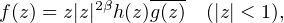from the unit disk D to the complex plane , where h and g are analytic in the unit disk, g(0) = 1 and Re{β} > -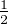. Furthermore, we obtain some necessary conditions for a function to be normal.

Ji Guo Institute of Mathematics, Academia Sinica, 6F, Astronomy-Mathematics Building, No. 1, Sec. 4, Roosevelt Road, Taipei 10617, Taiwan (jiguo@gate.sinica.edu.tw).
Quotient problem for entire functions with moving targets, pp. 627–650
ABSTRACT. As an analogue of the Hadamard quotient problem in number theory, the quotient problem (in the sense of complex entire functions) for two sequences of entire functions has recently been solved by Guo. In this paper, we consider the generalization of this problem in which we allow the coefficients to be entire functions of small growth by modifying the second main theorem with moving targets to a truncated version. We also compare our result to a special case in exponential polynomials first studied by Ritt.

Armando Corro Instituto de Matemática e Estatística, Universidade Federal de Goiás - UFG, 74690-900, Goiânia, GO, Brazil (corro@ufg.br), Marcelo Souza Instituto de Matemática e Estatística, Universidade Federal de Goiás - UFG, 74690-900, Goiânia, GO, Brazil (msouza@ufg.br), and Romildo Pina Instituto de Matemática e Estatística, Universidade Federal de Goiás - UFG, 74690-900, Goiânia, GO, Brazil (romildo@ufg.br)
Classes of Weingarten surfaces in S2 × , pp. 651–664
ABSTRACT. In this study, we explore surfaces in radial conformally flat 3-spaces. We characterize some specific surfaces of rotation with constant Gaussian and extrinsic curvatures in these radial 3-spaces. We obtain a special conformal space that is isometric to S2 × . We denote this space by M3 and refer to it as the radial model of S2 × . We study two classes of Weingarten surfaces in M3. The first class satisfies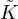E +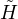2 -= 0, where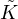is the Gaussian curvature,is the mean curvature, andE is the extrinsic curvature. We prove that each surface S of this class in M3 corresponds to a minimal surface in 3 or S corresponds to the inversion of a minimal surface in 3. The second class satisfies 2E -= 0, and we prove that each surface in this class in M3 corresponds to the EDSGW-surfaces (i.e., surfaces X satisfying the relation 2X,NH + X,XK = 0, where N is the unit normal of X) in the Euclidean space 3. Consequently, each of these two classes of surfaces has a Weierstrass-type representation depending on two holomorphic functions.

Ewa Kozłowska-Walania Institute of Mathematics, Faculty of Mathematics, Physics and Informatics, University of Gdańsk, Wita Stwosza 57, 80-952 Gdańsk, Poland (retrakt@mat.ug.edu.pl)
Real equations for Riemann surfaces admitting an extremal configuration of three symmetries, pp. 665–679
ABSTRACT. We consider all the Riemann surfaces with an extremal configuration of three symmetries, that is the ones with the maximal total number of ovals, being 2g + 4 for g odd and 2g + 3 for g even. It is known that in such a case the symmetries commute. We find all the corresponding equations for the underlying complex algebraic curve and its real forms.

Juan de Dios Pérez Juan de Dios Pérez Departamento de Geometría y Topología, Universidad de Granada, 18071, Granada, Spain (jdperez@ugr.es), Doo Hyun Hwang Department of Mathematics and RIRCM, Kyungpook National University, Daegu, 41566, Korea (engus0322@knu.ac.kr), and Young Jin Suh Department of Mathematics and RIRCM, Kyungpook National University, Daegu, 41566, Korea (yjsuh@knu.ac.kr)
Hypersurfaces in complex Grassmannians whose geodesics are circles and straight lines, pp. 681–693
ABSTRACT. In this paper we give a new geometric characterization of real hypersurfaces of type (A) in a complex two-plane Grassmannian G2(m+2), that is, a tube over a totally geodesic G2(m+1) in G2(m+2) by observing geodesics which are circles or straight lines in G2(m+2).

Jia Xie School of Mathematics and Information Science, Guangzhou University, Guangzhou 510006, China. (zyb-may@qq.com), Yongyi Gu Big data and Educational Statistics Application Laboratory, Guangdong University of Finance and Economics, Guangzhou 510320, China. (gdguyongyi@163.com), and Wenjun Yuan School of Mathematics and Information Science, Guangzhou University, Guangzhou 510006, China. (Corresponding author: wjyuan1957@126.com)
Entire solutions of a certain type of nonlinear differential equations, pp. 695–703
ABSTRACT. In this paper, we study the entire solutions of the differential equations was considered by Li. Moreover, we use another method to improve some results of Chen and Gao, whose condition “finite order” can be omitted.

Richard Lechner Institute of Analysis, Johannes Kepler University Linz, Altenberger Strasse 69, A-4040 Linz, Austria (Richard.Lechner@jku.at)
Direct sums of finite dimensional SLn spaces, pp. 705–745
ABSTRACT. SL denotes the space of functions whose square function is in L, and the subspaces SLn, n , are the finite dimensional building blocks of SL. We show that the identity operator IdSLn on SLn well factors through operators T : SLNSLN having large diagonal with respect to the standard Haar system. Moreover, we prove that IdSLn well factors either through any given operator T : SLNSLN, or through IdSLN- T. Let X(r) denote the direct sumn0SLnr, where 1 r ≤∞. Using Bourgain’s localization method, we obtain from the finite dimensional factorization result that for each 1 r ≤∞, the identity operator IdX(r) on X(r) factors either through any given operator T : X(r) X(r), or through IdX(r) - T. Consequently, the spacesn0SLnr, 1 r ≤∞ are all primary.

Bernhard Burgstaller Departamento de Matematica, Universidade Federal de Santa Catarina, CEP 88.040-900 Florianópolis-SC, Brasil (bernhardburgstaller@yahoo.de)
A note on a certain Baum–Connes map for inverse semigroups, pp. 747–769
ABSTRACT. Let G denote a countable inverse semigroup. We construct a kind of a Baum–Connes map K(Ã G) K(A G) by a categorial approach via localization of triangulated categories, developed by R. Meyer and R. Nest for groups G. We allow the coefficient algebras A to be in a special class of algebras called fibered G-algebras. This note continues and fixes our preprint “Attempts to define a Baum–Connes map via localization of categories for inverse semigroups”.

Shuo Zhang School of Mathematical Sciences, Shanghai Jiao Tong University, 800 Dong Chuan Road, Shanghai, 200240, P.R. China. (zs.math@sjtu.edu.cn)
Lp-norm estimate for the Bergman projections, pp. 771–796
ABSTRACT. We study the Lp-norm estimates for the Bergman projection operators on several types of generalized Hartogs triangles in this note. As a direct application of our main results, we obtain the asymptotic behaviors for the Lp-norm of the Bergman projections when p tends to the endpoints of the range of p in which the Bergman projection operators are bounded on Lp spaces.

S. Berhanu Department of Mathematics, Temple University, Philadelphia, PA 19122, USA (berhanu@temple.edu) and Jemal Yesuf Department of Mathematics, Addis Ababa University, Ethiopia (jemalyesuf@su.edu.et)
Continuity of a class of FBI transforms on Sobolev spaces, pp. 797–808
ABSTRACT. We show that a subclass of the generalized FBI transforms that were introduced in prior work are bounded on Sobolev spaces.

V.V. Tkachuk, Departamento de Matemáticas, Universidad Autónoma Metropolitana, Av. San Rafael Atlixco, 186, Col. Vicentina, Iztapalapa C.P. 09340, Mexico City, Mexico. Current address: 221 Parker Hall, Department of Mathematics and Statistics, Auburn University, Auburn, AL, 36849 U.S.A. (vova@xanum.uam.mx)
The extent of a weakly exponentially separable space can be arbitrarily large, pp. 809–819
ABSTRACT. We prove that a weakly exponentially separable space has extent not exceeding 𝔠 if it is either normal or first countable. Any metalindelöf weakly exponentially separable space must be Lindelöf. Besides, for any infinite cardinal κ, there exists a weakly exponentially separable space X with ext(X) = κ; this result solves a published open question.

Norberto Ordoñez Universidad Autónoma del Estado de México, Facultad de Ciencias, Instituto Literario No. 100, Col. Centro, Toluca, Estado de México, C. P. 50000, MÉXICO (nordonezr@uaemex.mx)
The hyperspace of meager subcontinua, pp. 821–834
ABSTRACT. Given a metric continuum X, we define the hyperspace of meager subcontinua of X, denoted by M(X), as the collection of all subcontinua of X with empty interior. In this paper we study the connectedness, compactness, arcwise connectedness and contractibility of this hyperspace. As a main result we obtain that for a smooth dendroid X the hyperspace M(X) is contractible. Also we raise open problems.

B. D. Daniel Lamar University, Department of Mathematics, Beaumont, Texas 77710-0047 (dale.daniel@lamar.edu) and M. Tuncali Nipissing University, Department of Computer Science and Mathematics, North Bay, Ontario P1B 8L7 (muratt@nipissingu.ca)
Metrizability of products in compacta in which each continuum of convergence is metrizable, pp. 835–843
ABSTRACT. Treybig showed that if the product of two infinite compacta is the image of some compact ordered space then each factor of the product must be metrizable. Treybig’s theorem motivates the study of those conditions under which a space admits products with only metric components. Somewhat later, the second-named author extended this result to continua satisfying various rim properties. A natural condition to impose on such spaces is that the space have the property that each continuum of convergence is metrizable. Herein, we give some product theorems in such spaces.